Question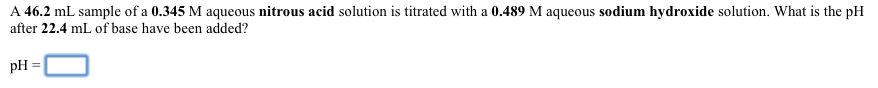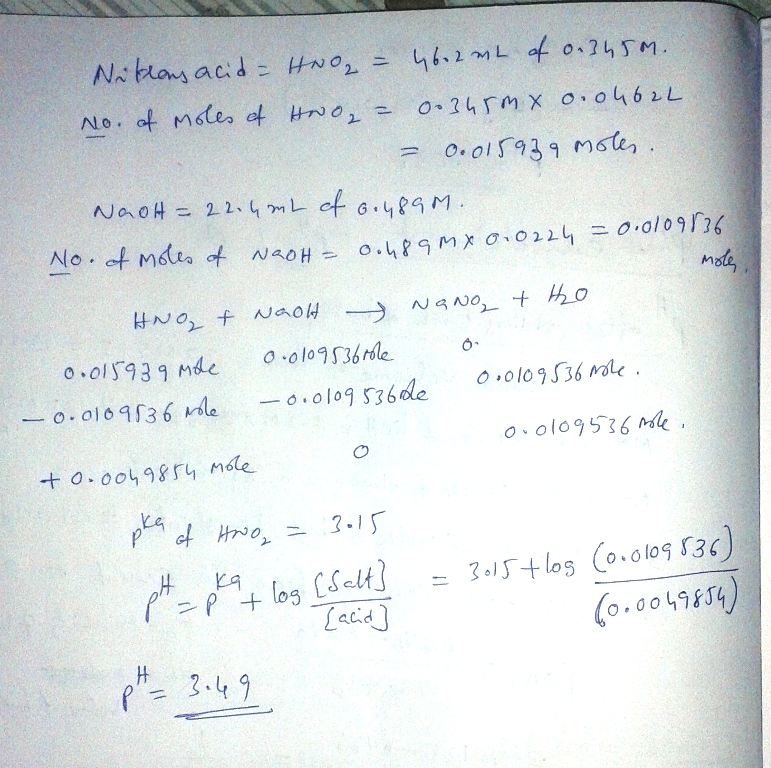#### Earn Coins

Coins can be redeemed for fabulous gifts.

Similar Homework Help Questions
• ### When a 26.7 ml sample of a 0.361 M aqueous nitrous acid solution is titrated with...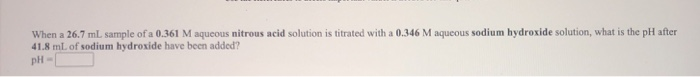When a 26.7 ml sample of a 0.361 M aqueous nitrous acid solution is titrated with a 0.346 M aqueous sodium hydroxide solution, what is the pH after 41.8 mL of sodium hydroxide have been added? pH- A 47.8 ml sample of a 0.422 M aqueous nitrous acid solution is titrated with a 0.473 M aqueous sodium hydroxide solution. What is the pH after 17.2 mL of base have been added? pH-

• ### (1) A 31.4 mL sample of a 0.531 M aqueous nitrous acid solution is titrated with...

(1) A 31.4 mL sample of a 0.531 M aqueous nitrous acid solution is titrated with a 0.305 M aqueous barium hydroxide solution. What is the pH after 10.4 mL of base have been added? (2) When a 28.7 mL sample of a 0.424 M aqueous hydrofluoric acid solution is titrated with a 0.352 M aqueous sodium hydroxide solution, what is the pH after 51.9 mL of sodium hydroxide have been added?

• ### When a 23.2 mL sample of a 0.345 M aqueous acetic acid solution is titrated with...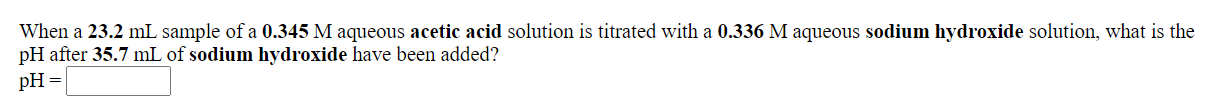When a 23.2 mL sample of a 0.345 M aqueous acetic acid solution is titrated with a 0.336 M aqueous sodium hydroxide solution, what is the pH after 35.7 mL of sodium hydroxide have been added? pH = What is the pH at the equivalence point in the titration of a 27.6 mL sample of a 0.423 M aqueous hydrocyanic acid solution with a 0.431 M aqueous potassium hydroxide solution? pH=

• ### When a 28.9 mL sample of a 0.453 M aqueous nitrous acid solution is titrated with...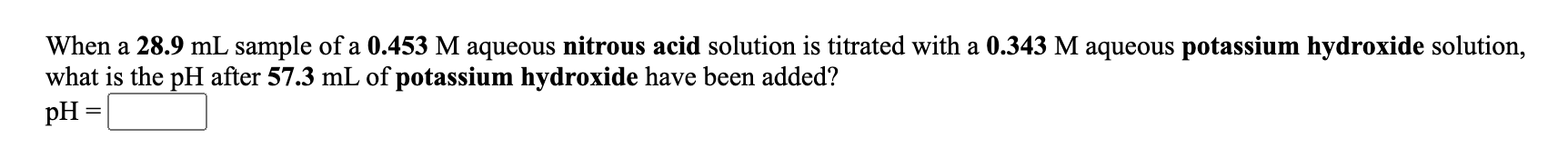When a 28.9 mL sample of a 0.453 M aqueous nitrous acid solution is titrated with a 0.343 M aqueous potassium hydroxide solution, what is the pH after 57.3 mL of potassium hydroxide have been added? When a 28.9 mL sample of a 0.453 M aqueous nitrous acid solution is titrated with a 0.343 M aqueous potassium hydroxide solution, what is the pH after 57.3 mL of potassium hydroxide have been added? pH =

• ### A 38.9 mL sample of a 0.486 M aqueous nitrous acid solution is titrated with a...

A 38.9 mL sample of a 0.486 M aqueous nitrous acid solution is titrated with a 0.400 M aqueous barium hydroxide solution. What is the pH after 7.85 mL of base have been added? pH =

• ### 28) When a 21.7 mL sample of a 0.378 M aqueous nitrous acid solution is titrated...

28) When a 21.7 mL sample of a 0.378 M aqueous nitrous acid solution is titrated with a 0.496 M aqueous sodium hydroxide solution, what is the pH after 24.8 mL of sodium hydroxide have been added? pH = ___

• ### When a 25.9 mL sample of a 0.317 M aqueous nitrous acid solution is titrated with...

When a 25.9 mL sample of a 0.317 M aqueous nitrous acid solution is titrated with a 0.458 M aqueous sodium hydroxide solution, what is the pH after 26.9 mL of sodium hydroxide have been added? Please show work!

• ### 1.When a 15.1 mL sample of a 0.479 M aqueous nitrous acid solution is titrated with...

1.When a 15.1 mL sample of a 0.479 M aqueous nitrous acid solution is titrated with a 0.483 M aqueous sodium hydroxide solution, what is the pH after 22.5 mL of sodium hydroxide have been added? 2.A 21.6 mL sample of 0.214 M diethylamine, (C2H5)2NH, is titrated with 0.256 M perchloric acid. The pH before the addition of any perchloric acid is

• ### When a 23.3 mL sample of a 0.443 M aqueous nitrous acid solution is titrated with...

When a 23.3 mL sample of a 0.443 M aqueous nitrous acid solution is titrated with a 0.305 M aqueous barium hydroxide solution, what is the pH after 25.4 mL of barium hydroxide have been added? pH =

• ### When a 23.8 mL sample of a 0.443 M aqueous hydrofluorie acid solution is titrated with...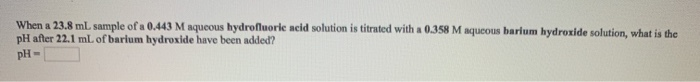When a 23.8 mL sample of a 0.443 M aqueous hydrofluorie acid solution is titrated with a 0.358 M aqueous barium hydroxide solution, what is the pH after 22.1 mL of barium hydroxide have been added? pH- What is the pH at the equivalence point in the titration of a 19.7 mL sample of a 0.376 M aqueous nitrous acid solution with a 0.447 M aqueous sodium hydroxide solution? pH- When a 20.1 mL sample of a 0.417 M aqueous...